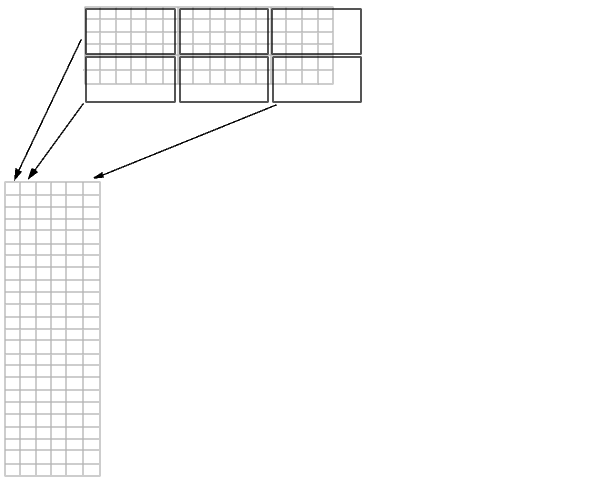Image Processing Toolbox User's GuideDistinct Blocks

For a distinct block operation, `colfilt` creates a temporary matrix by rearranging each block in the image into a column. `colfilt` pads the original image with 0's, if necessary, before creating the temporary matrix.

The following figure illustrates this process. A 6-by-16 image matrix is processed in 4-by-6 blocks. `colfilt` first zero-pads the image to make the size 8-by-18 (six 4-by-6 blocks), and then rearranges the blocks into six columns of 24 elements each.colfilt Creates a Temporary Matrix for Distinct Block Operation

After rearranging the image into a temporary matrix, `colfilt` passes this matrix to the function. The function must return a matrix of the same size as the temporary matrix. If the block size is `m`-by-`n`, and the image is `mm`-by-`nn`, the size of the temporary matrix is `(m*n)`-by-(`ceil(mm/m)*ceil(nn/n))`. After the function processes the temporary matrix, the output is rearranged into the shape of the original image matrix.

This example sets all the pixels in each 8-by-8 block of an image to the mean pixel value for the block, producing the same result as the `blkproc` example in Distinct Block Operations.

• ```I = im2double(imread('tire.tif'));
f = inline('ones(64,1)*mean(x)');
I2 = colfilt(I,[8 8],'distinct',f);
```

Notice that the inline function computes the mean of the block and then multiplies the result by a vector of ones, so that the output block is the same size as the input block. As a result, the output image is the same size as the input image.

Restrictions

You can use `colfilt` to implement many of the same distinct block operations that `blkproc` performs. However, `colfilt` has certain restrictions that `blkproc` does not:

• The output image must be the same size as the input image.
• The blocks cannot overlap.

For situations that do not satisfy these constraints, use `blkproc`.Sliding Neighborhoods Function Reference